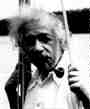### Math 712. Topics in Mathematical Physics: General Relativity - Spring 18 - Hans Lindblad

We will show that the initial value problem for Einstein's equations has a local unique solution (in harmonic coordinates). We will also show that Einstein's equations have global solutions (in harmonic coordinates) if initial conditions are close to flat space, as in my recent work with Rodnianski. Einstein's equation in harmonic coordinates become a system of nonlinear wave equations. We will therefore develop the tools needed to show existence and estimates for nonlinear wave equations. Long time existence for nonlinear wave equations will therefore be the starting point of theses seminars. A good place to read about this is Chapter 6 in Hormander's book 'Lectures on Nonlinear Hyperbolic Differential Euqations'. A more geometric text is Alinhac's book 'Geometric Analysis of Hyperbolic Equations an introduction'. A place to learn some of the techniques for simple nonlinear wave equations is my paper Lindblad. My paper(s) about global existence for Einstein's equations can be found at arXiv See in particular the original papers with Rodnianski and a recent simplifications with Taylor and Tohaneanu. The course is assuming that you know local existence for nonlinear wave equations as in Evans PDE book Chapter 7 that is taught in the second part of the graduate PDE sequence Math 632 PDE.

It is also useful to first read a physics undergraduate/graduate text book like Carroll "Spacetime and Geometry".

The lectures are MW 3-4 in Krieger 406 or the chair's office.